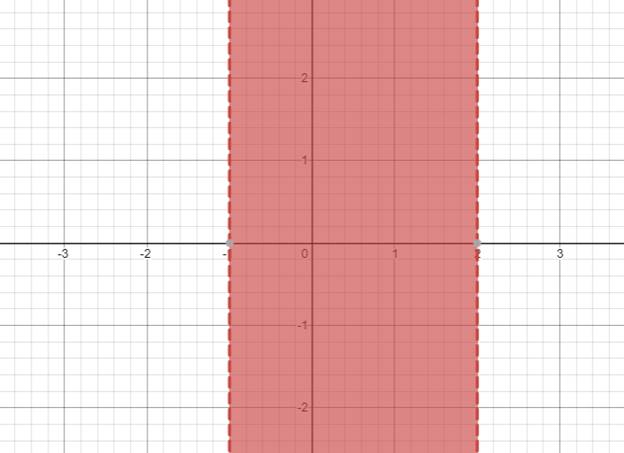# The solution of given nonlinear inequality equation and plot a graph for it.### Precalculus: Mathematics for Calcu...

6th Edition
Stewart + 5 others
Publisher: Cengage Learning
ISBN: 9780840068071### Precalculus: Mathematics for Calcu...

6th Edition
Stewart + 5 others
Publisher: Cengage Learning
ISBN: 9780840068071

#### Solutions

Chapter 1.7, Problem 42E
To determine

## The solution of given nonlinear inequality equation and plot a graph for it.

Expert Solution

1<x<2

### Explanation of Solution

Given:

Non-linear inequality equation

x2<x+2

Calculation,

Non-linear inequality equation,

x2<x+2x2x2>0x2(21)x2>0x22x+x2>0x(x2)+1(x2)>0(x+1)(x2)>0Now,(x+1)>0x>1and(x2)<0x<2so,1<x<2

Now, graph of x2<x+2Conclusion:

Hence, the values of x lies between the -1 and 2.

### Have a homework question?

Subscribe to bartleby learn! Ask subject matter experts 30 homework questions each month. Plus, you’ll have access to millions of step-by-step textbook answers!